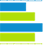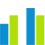# Chart with two value axesBar chart with two value axesCol. chart with two value axes

A bar (column) chart with two value axes compares two measures across one or two dimensions. The measures represent mixed types of data that cannot be compared with each other because they relate to a different scale or different units of measurement.

## When to use

The bar (column) chart with two value axes can be used to show the following aspects:

• The vehicle's horsepower and MPG (miles per gallon) by vehicle type.
• The average monthly rainfall and temperature by location or month.
• The total price and the sum of units purchased by year.

If the axes use the same scale or relate to the same units of measurement, it can be a good idea to use a column and line chart instead. For details, see Bar (column) and line chart.

## Data requirements

To build this chart, define the data fields as follows:

• Dimension – One or two dimensions. The order of the dimensions matters. The first dimension represents the main grouping option.
• Measure – Two measures
• Tooltip – (Optional) One or more measures
• Trellis – (Optional) One dimension
• Color – (Optional) One dimension

## Use case

View the following use cases for the chart with two value axes, based on the chart orientation.

## References

For details on how to customize your visualization, see Visualization settings.

For a whole list of visualizations, see the following topics: